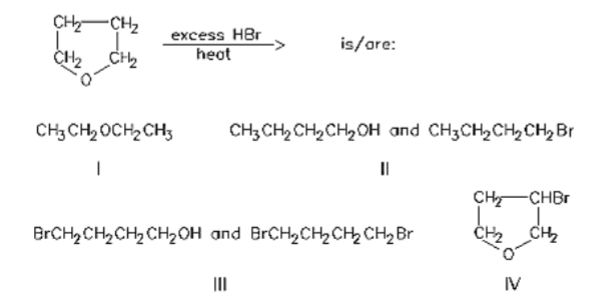# Problem: Predict the product for the following reaction and determine if the product is given in the answer options below. If the answer is given, select the answer. Write "none" if the answer is not given.

###### FREE Expert Solution
85% (433 ratings)
###### Problem Details

Predict the product for the following reaction and determine if the product is given in the answer options below. If the answer is given, select the answer. Write "none" if the answer is not given.What scientific concept do you need to know in order to solve this problem?

Our tutors have indicated that to solve this problem you will need to apply the Ether Cleavage concept. You can view video lessons to learn Ether Cleavage. Or if you need more Ether Cleavage practice, you can also practice Ether Cleavage practice problems.

What is the difficulty of this problem?

Our tutors rated the difficulty ofPredict the product for the following reaction and determine...as medium difficulty.

How long does this problem take to solve?

Our expert Organic tutor, Chris took 5 minutes and 30 seconds to solve this problem. You can follow their steps in the video explanation above.

What professor is this problem relevant for?

Based on our data, we think this problem is relevant for Professor Bertrand's class at UCSD.